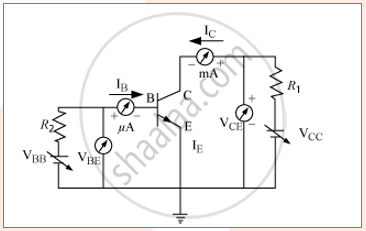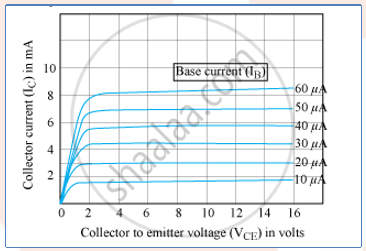# Draw the Circuit Arrangement for Studying the Output Characteristics of N-p-n Transistor in Ce Configuration. Explain How the Output Characteristics Are Obtained. - Physics

Draw the circuit arrangement for studying the output characteristics of n-p-n transistor in CE configuration. Explain how the output characteristics are obtained.

#### Solution

Circuit arrangement for studying the output characteristics of n-p-n transistor in CE configurationVariation of collector current I_c with the collector-emitter voltage V_(CE) is called output characteristic.The output characteristics describe the relationship between output current (I_c) and output voltage (V_(cE)). First, draw a vertical line and a horizontal line. The vertical line represents the y-axis and the horizontal line represents the x-axis. The output current or collector current (Ic) is taken along the y-axis (vertical line) and the output voltage (V_(cE)) is taken along the x-axis (horizontal line). To determine the output characteristics, the input current or base current 18 is kept constant at 0 pA and the output voltage V_(cE) is increased from zero volts to different voltage levels. For each level of the output voltage, the corresponding output current (Ic) is recorded. A curve is then drawn between output current Ic and output voltage V_(cE) at constant input current 18 (0 pA).

Concept: Transistor Action
Is there an error in this question or solution?